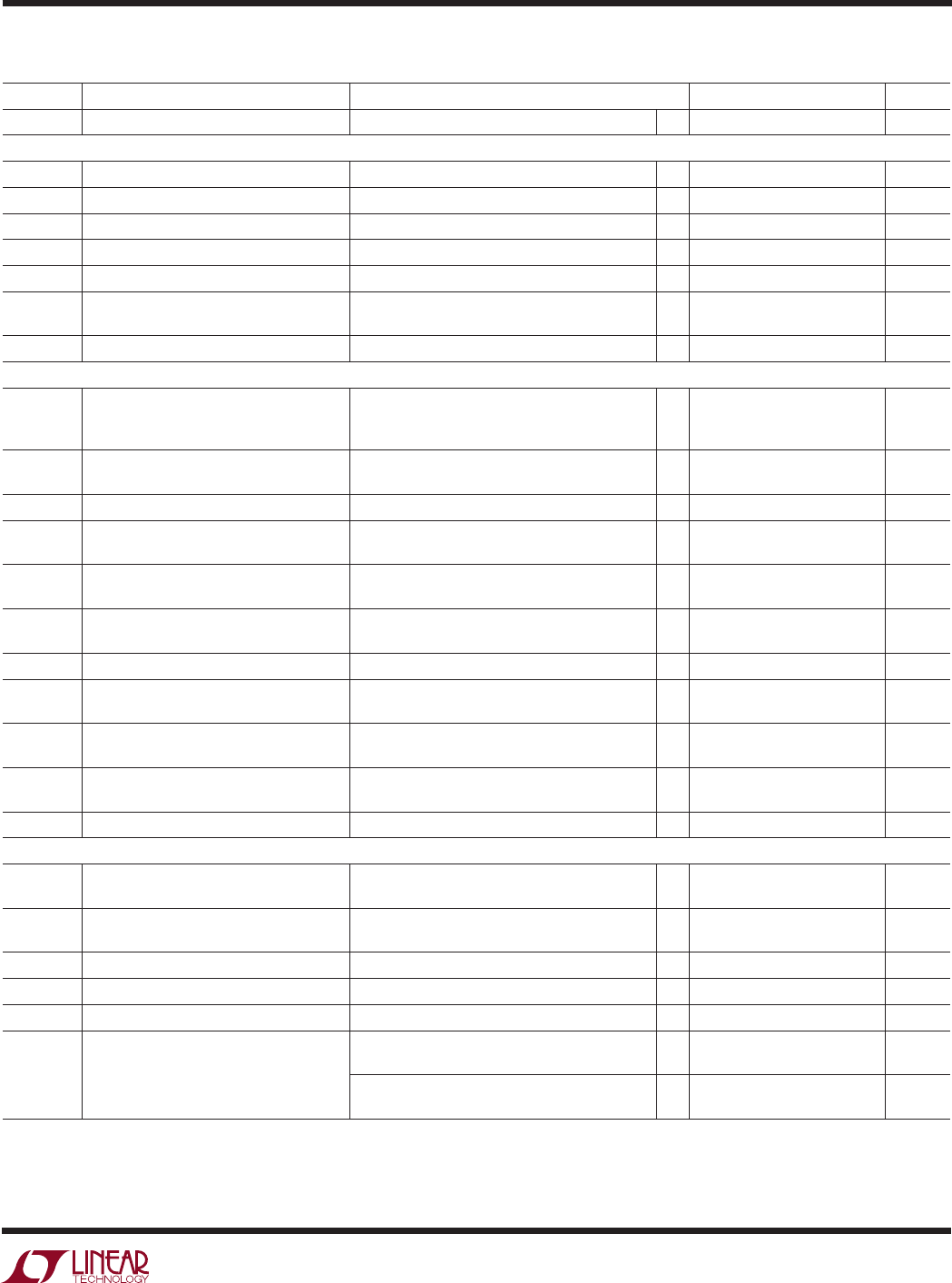# Datasheet3
LTC1343
ELECTRICAL CHARACTERISTICS
The denotes specifications which apply over the full operating
temperature range, otherwise specifications are at T
A
= 25°C. V
CC
= 5V (Notes 2, 3)
SYMBOL PARAMETER CONDITIONS MIN TYP MAX UNITS
t
r
Supply Rise Time No-Cable Mode or Power-Up to Turn On 2 ms
Logic Inputs and Outputs
V
IH
Logic Input High Voltage 2V
V
IL
Logic Input Low Voltage 0.8 V
I
IN
Logic Input Current ±10 µA
V
OH
Output High Voltage I
O
= –4mA 3 4.5 V
V
OL
Output Low Voltage I
O
= 4mA 0.3 0.8 V
I
OSR
Output Short-Circuit Current 0V V
O
V
CC
, 0°C T
A
70°C –60 60 mA
0V V
O
V
CC
, –40°C T
A
85°C –70 70 mA
I
OZR
Three-State Output Current M0 = M1 = M2 = V
CC
, 0V V
O
V
CC
±1 µA
V.11 Driver
V
OD
Differential Output Voltage Open Circuit, R
L
= 1.95k ±6V
R
L
= 50 (Figure 1), ±2V
V
OD
at 50 > 1/2 V
OD
at R
L
= 1.95k
V
OD
Change in Magnitude of Differential R
L
= 50 (Figure 1) 0.2 V
Output Voltage
V
OC
Common Mode Output Voltage R
L
= 50 (Figure 1) 3.0 V
V
OC
Change in Magnitude of Common Mode R
L
= 50 (Figure 1) 0.2 V
Output Voltage
I
SS
Short-Circuit Current 0.25V V
O
0.25V, Power Off or ±150 mA
No-Cable Mode or Driver Disabled
I
OZ
Output Leakage Current 0.25V V
O
0.25V, Power Off or ±0.01 ±100 µA
No-Cable Mode or Driver Disabled
t
r
, t
f
Rise or Fall Time (Figures 2, 6) 41325 ns
t
PLH
Input to Output (Figures 2, 6), 0°C T
A
70°C 25 55 80 ns
(Figures 2, 6), –40°C T
A
85°C 25 55 90 ns
t
PHL
Input to Output (Figures 2, 6), 0°C T
A
70°C 25 55 80 ns
(Figures 2, 6), –40°C T
A
85°C 25 55 90 ns
t Input to Output Difference, t
PLH
– t
PHL
(Figures 2, 6), 0°C T
A
70°C 0317 ns
(Figures 2, 6), –40°C T
A
85°C 0325 ns
t
SKEW
Output to Output Skew (Figures 2, 6) 3 ns
V
TH
Input Threshold Voltage 7V V
CM
7V, 0°C T
A
70°C 0.2 0.2 V
–7V V
CM
7V, –40°C T
A
85°C 0.3 0.3 V
V
TH
Input Hysteresis 7V V
CM
7V, 0°C T
A
70°C 15 40 mV
–7V V
CM
7V, –40°C T
A
85°C 60 mV
I
IN
Input Current (A, B) 10V V
A, B
10V ±0.50 mA
R
IN
Input Impedance 10V V
A, B
10V 20 32 k
t
r
, t
f
Rise or Fall Time (Figures 2, 7) 15 ns
t
PLH
Input to Output (Figures 2, 7), CTRL = GND, 0°C T
A
70°C 35 80 115 ns
CTRL = V
CC
, 0°C T
A
70°C 400 ns
(Figures 2, 7), CTRL = GND, –40°C T
A
85°C 25 80 130 ns
CTRL = V
CC
, –40°C T
A
85°C 400 ns